﻿ 理论、实操慕课课程用户翘课影响因素研究 Research on the Influencing Factors of User’s Dropout in Theory and Practice Class

Computer Science and Application
Vol. 09  No. 11 ( 2019 ), Article ID: 33013 , 13 pages
10.12677/CSA.2019.911231

Research on the Influencing Factors of User’s Dropout in Theory and Practice Class

Mengjing Qi1, Mingxian Wang2, Lei La1

1School of Information Technology & Management, University of International Business and Economics, Beijing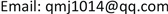Received: Oct. 30th, 2019; accepted: Nov. 12th, 2019; published: Nov. 19th, 2019ABSTRACT

Due to the high skipping rate and low completion rate of MOOCs, it is difficult for MOOCs to make full use of the advantages of Shared service economy to promote the reform of China’s education model. Current scholars’ prediction of skipping MOOCs has some problems, such as low accuracy and lack of targeted analysis of different courses. Select new feature variables and use XGBoost algorithm in integrated learning to model and predict the background data of different types of courses. Therefore, the factors influencing students’ skipping classes of different types of courses are studied through comparison. The experimental results are compared with the traditional machine learning algorithm SVM, logistic regression and integrated learning algorithm AdaBoost. The research proves the effectiveness of XGBoost algorithm in the prediction of skipping MOOCs, and the difference of influencing factors of different types of courses. The results are of great practical significance to the improvement of platform retention rate and effective utilization rate of resources.

Keywords:Moocs, Prediction of Dropout, XGBoost

1对外经济贸易大学信息学院，北京

2对外经济贸易大学教务处，北京1. 引言

XGBoost  是一种基于梯度提升决策树的集成学习算法。一经提出便在众多竞赛项目里大放异彩。XGBoost因为出色的学习效果、快速的迭代速度以及可并行性等优势受到广泛关注并很快在各大领域得以应用。

2. 数据预处理

2.1. 数据来源

2.2. 特征选择

Table 1. Description of factors

2.3. 数据预处理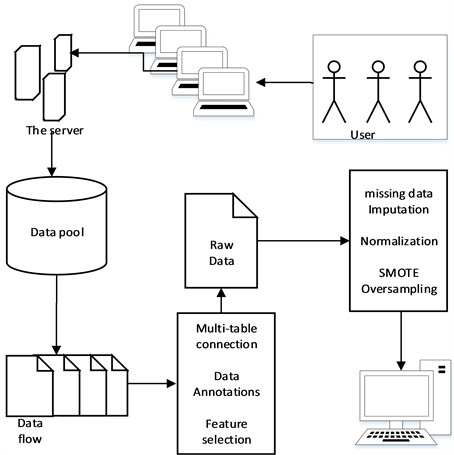Figure 1. Data preprocessing schematic

1) 特征选取、计算及数据标注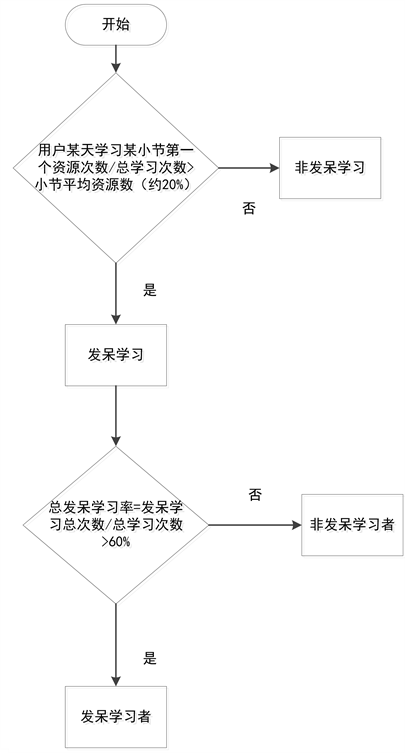Figure 2. Student’s dashed behavior discrimination flow chart

2) 缺失值填充

3) 归一化处理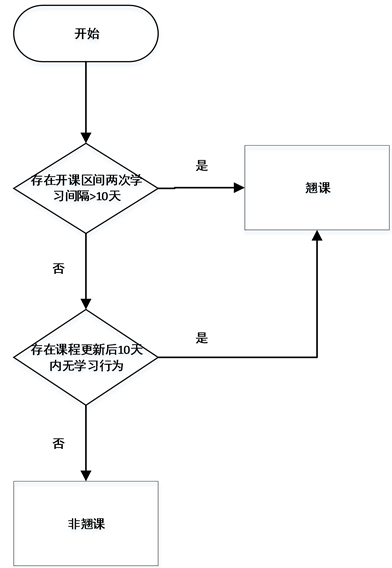Figure 3. Student skipping behavior discriminating flow chart

4) 非平衡化处理

3. 基于XGBoost的翘课预测

3.1. XGBoost算法

$J\left(\theta \right)=\underset{i=1}{\overset{n}{\sum }}l\left({y}_{i},{\stackrel{^}{y}}_{t}\right)+\underset{k=1}{\overset{K}{\sum }}\Omega \left({f}_{k}\right)$ (1)

XGBoost为增量训练，即在前一步的基础之上新增一棵树，并优化新增树。

$\begin{array}{l}{\stackrel{^}{y}}_{i}^{\left(0\right)}=0\\ {\stackrel{^}{y}}_{i}^{\left(1\right)}={f}_{1}\left({x}_{i}\right)={\stackrel{^}{y}}_{i}^{\left(0\right)}+{f}_{1}\left({x}_{i}\right)\\ {\stackrel{^}{y}}_{i}^{\left(2\right)}={f}_{1}\left({x}_{i}\right)+{f}_{2}\left({x}_{i}\right)={\stackrel{^}{y}}_{i}^{\left(1\right)}+{f}_{2}\left({x}_{i}\right)\\ \text{\hspace{0.17em}}\text{\hspace{0.17em}}\text{\hspace{0.17em}}\text{\hspace{0.17em}}\text{\hspace{0.17em}}\text{ }\text{\hspace{0.17em}}⋮\\ {\stackrel{^}{y}}_{i}^{\left(t\right)}=\underset{k=1}{\overset{t}{\sum }}{f}_{k}\left({x}_{i}\right)={\stackrel{^}{y}}_{i}^{\left(t-1\right)}+{f}_{t}\left({x}_{i}\right)\end{array}$ (2)

${\stackrel{^}{J}}_{i}^{\left(t\right)}=\underset{i=1}{\overset{n}{\sum }}l\left({y}_{i},{\stackrel{^}{y}}_{i}^{\left(t-1\right)}+{f}_{t}\left({x}_{i}\right)\right)+\Omega \left({f}_{t}\right)$ (3)

${J}^{\left(t\right)}=\underset{i=1}{\overset{n}{\sum }}l\left({y}_{i},{\stackrel{^}{y}}_{i}^{\left(t-1\right)}+{g}_{i}{f}_{t}\left({x}_{i}\right)+\frac{1}{2}{h}_{i}{f}_{t}^{2}\left({x}_{i}\right)\right)+\Omega \left({f}_{t}\right)$ (4)

${J}^{\left(t\right)}=\underset{i=1}{\overset{n}{\sum }}l\left({g}_{i}{f}_{t}\left({x}_{i}\right)\right)+\frac{1}{2}{h}_{i}{f}_{t}^{2}\left({x}_{i}\right)+\Omega \left({f}_{t}\right)$ (5)

$\Omega \left(f\right)=\gamma T+\frac{1}{2}\lambda \underset{j=1}{\overset{T}{\sum }}{w}_{j}^{2}$ (6)

${J}^{\left(t\right)}=\underset{j=1}{\overset{T}{\sum }}\left[{G}_{j}{w}_{j}+\frac{1}{2}\left({H}_{j}+\lambda \right){w}_{j}^{2}\right]+\gamma T$ (7)

${w}_{j}$ 求导可得，

${w}_{j}^{\ast }=-\frac{{G}_{j}}{{H}_{j}+\lambda }$ (8)

${J}^{\ast }=-\frac{1}{2}\underset{j=1}{\overset{T}{\sum }}\frac{{G}_{j}^{2}}{{H}_{j}+\lambda }+\gamma T$(9)

3.2. 评价标准

$P=\frac{\text{TP}}{\text{TP}+\text{FP}}$ (10)

$R=\frac{\text{TP}}{\text{TP}+\text{FN}}$ (11)

F1：精确率与召回率的调和平均值：

$\text{F}1=\frac{2\ast P\ast R}{P+R}$ (12)

$A=\frac{\text{TP}+\text{TN}}{\text{TP}+\text{TN}+\text{FP}+\text{FN}}$ (13)

AUC (Area under curve)：M为正样本个数，N为负样本个数时

$\text{AUC}=\frac{{\sum }_{正样本}rank\left(score\right)-\frac{M\ast \left(M+1\right)}{2}}{M\ast N}$ (14)

3.3. 实验流程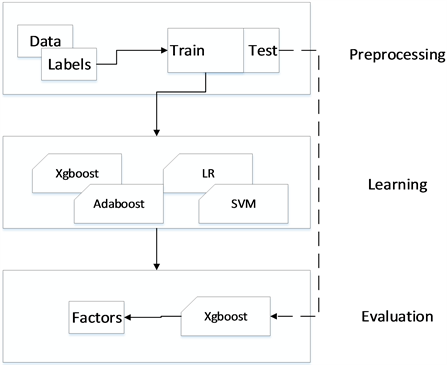Figure 4. Experimental flow diagram

4. 实验与结果分析

4.1. 实验Table 3. Comparison of Different Models in “Interactive Whiteboard Teaching Application”Table 4. Comparison of different models of “How do teachers do research”Table 5. The effect after adjusting the parameters of XGboost

4.2. 结果分析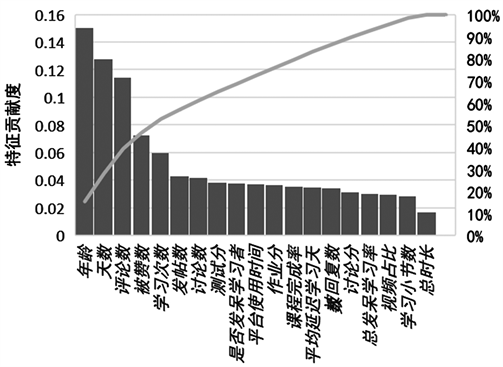Figure 5. Feature contribution of “How do teachers do research”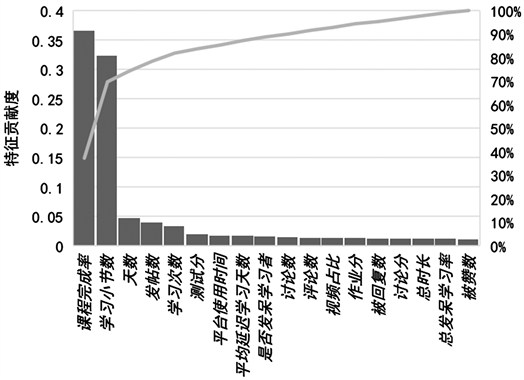Figure 6. Feature Contribution of “Interactive Whiteboard Teaching Application”

5. 结语

Research on the Influencing Factors of User’s Dropout in Theory and Practice Class[J]. 计算机科学与应用, 2019, 09(11): 2052-2064. https://doi.org/10.12677/CSA.2019.911231

1. 1. 富切尔•博茨曼, 路•罗杰斯. 共享经济时代: 互联网思维下的协同消费商业模式[M]. 唐朝文, 译. 上海: 上海交通大学出版社, 2015.

2. 2. Stein, L.A. (2012) Casting a Wider Net. Science, 338, 1422-1423. https://doi.org/10.1126/science.1230710

3. 3. Waldrop, M.M. (2013) Online Learning: Campus 2.0. Nature, 495, 160-163. https://doi.org/10.1038/495160a

4. 4. Yousef, A.M.F., Chatti, M.A., Schroeder, U., et al. (2014) What Drives a Successful MOOC? An Empirical Examination of Criteria to Assure Design Quality of MOOCs. 14th Interna-tional Conference on Advanced Learning Technologies, Athens, 7-10 July 2014, 44-48. https://doi.org/10.1109/ICALT.2014.23

5. 5. Jordan, K. (2014) Initial Trends in Enrolment and Completion of Massive Open Online Courses. International Review of Research in Open and Distance Learning, 15, 133-160. https://doi.org/10.19173/irrodl.v15i1.1651

6. 6. Hone, K.S. and Said, G.R.E. (2016) Exploring the Factors Affect-ing MOOC Retention: A Survey Study. Computers & Education, 98, 157-168. https://doi.org/10.1016/j.compedu.2016.03.016

7. 7. Chen, T.Q. and Guestrin, C. (2016) XGBoost: A Scalable Tree Boosting System. Proceedings of the 22nd ACM SIGKDD International Conference on Knowledge Discovery and Data Mining, San Francisco, 13-17 August 2016, 785-794. https://doi.org/10.1145/2939672.2939785

8. 8. 卢晓航, 王胜清, 黄俊杰, 陈文广, 闫增旺. 一种基于滑动窗口模型的MOOCs辍学率预测方法[J]. 数据分析与知识发现, 2017, 1(4): 67-75.

9. 9. 王雪宇, 邹刚, 李骁. 基于MOOC数据的学习者辍课预测研究[J]. 现代教育技术, 2017, 27(6): 94-100.

10. 10. Li, W., Gao, M., Li, H., et al. (2016) Dropout Prediction in MOOCs Using Behavior Features and Multi-View Semi-Supervised Learning. International Joint Conference on Neural Networks, Vancouver, 24-29 July 2016, 3130-3137. https://doi.org/10.1109/IJCNN.2016.7727598

11. 11. 叶倩怡, 饶泓, 姬名书. 基于XGBoost的商业销售预测[J]. 南昌大学学报(理科版), 2017, 41(3): 275-281.

12. 12. 崔艳鹏, 史科杏, 胡建伟. 基于XGBoost算法的Webshell检测方法研究[J]. 计算机科学, 2018, 45(S1): 375-379.

13. 13. 贺超凯, 吴蒙. edX平台教育大数据的学习行为分析与预测[J]. 中国远程教育, 2016(6): 54-59.

14. 14. Xing, W., Chen, X., Stein, J., et al. (2016) Temporal Predication of Dropouts in MOOCs: Reaching the Low Hanging Fruit through Stacking Generalization. Computers in Human Behavior, 58, 119-129. https://doi.org/10.1016/j.chb.2015.12.007

15. 15. Fei, M. and Yeung, D.Y. (2015) Temporal Models for Predicting Student Dropout in Massive Open Online Courses. IEEE International Conference on Data Mining Workshop, Atlantic City, 14-17 November 2015, 256-263. https://doi.org/10.1109/ICDMW.2015.174

16. 16. Chen, T. and Tong, H. (2014) Higgs Boson Discovery with Boosted Trees. International Conference on High-Energy Physics & Machine Learning, Montreal, 13 December 2014, 69-80.